Select Page

# CBSE Maths 12 Science Vector Algebra MCQ Solutions in English

CBSE Maths 12 Science Vector Algebra MCQ Solutions in English to enable students to get Solutions in a narrative video format for the specific question.

Expert Teacher provides CBSE Maths 12 Science Vector Algebra MCQ Solutions through Video Solutions in English language. This video solution will be useful for students to understand how to write an answer in exam in order to score more marks. This teacher uses a narrative style for a question from Vector Algebra not only to explain the proper method of answering question, but deriving right answer too.

Please find the question below and view the Solution in a narrative video format.

Question:

Solution Video in English:

You can select video Solutions from other languages also. Please check Solutions in ( Hindi )

## Similar Questions from CBSE, 12th Science, Maths, Vector Algebra

Question 1 :  P and Q are two points with position vectors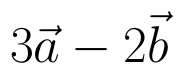and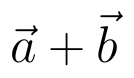respectively. Write the position vector of a point R which divides the line segment PQ externally in the ratio 2:1.  (View Answer Video)

Question 2 :  Find a vector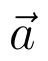of magnitude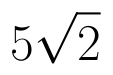making an angle of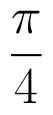with x-axis,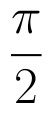with y-axis and an acute angle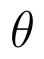with z-axis.  (View Answer Video)

Question 3 : Find the unit vector in the direction ofif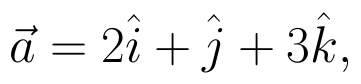and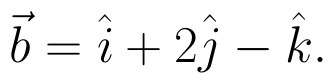(View Answer Video)

Question 4 : L and M are two points with position vectors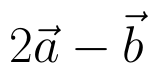and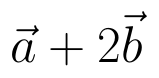respectively. Write the position vectors of a point N which divides the line segment LM in the ratio 2:1 externally.   (View Answer Video)

Question 5 : Find the value of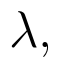if the points with position vectors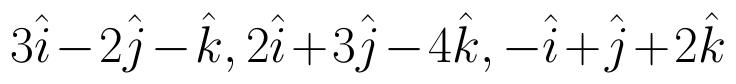and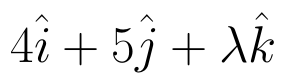are coplanar.  (View Answer Video)

### Integrals

Question 1 : Prove that :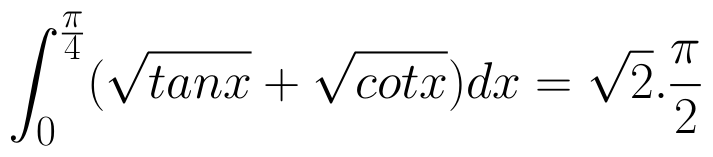(View Answer Video)

Question 2 : Evaluate :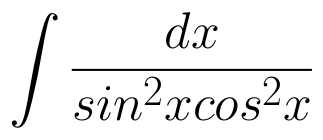(View Answer Video)

Question 3 :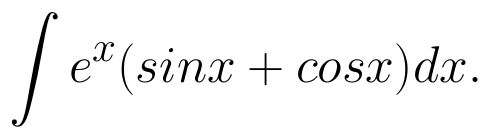(View Answer Video)

Question 4 : Evaluate the following :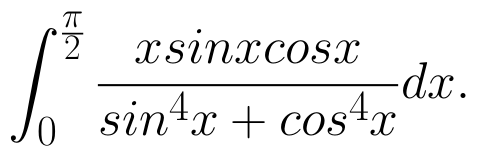(View Answer Video)

Question 5 : Write the value of :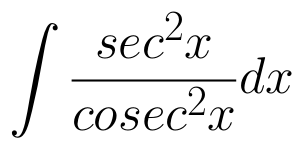(View Answer Video)

### Continuity and Differentiability

Question 1 : Differentiate w.r.t.x the function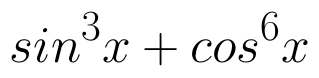. (View Answer Video)

Question 2 : If x and y are connected parametrically by the equation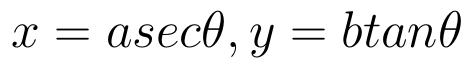, without eliminating the parameter, find. (View Answer Video)

Question 3 : Differentiate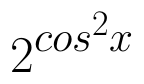w.r.t.x. (View Answer Video)

Question 4 : Findfor the function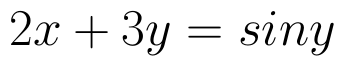. (View Answer Video)

Question 5 : If x and y are connected parametrically by the equation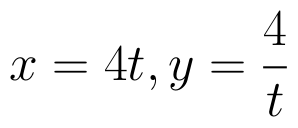, without eliminating the parameter, find. (View Answer Video)

### Determinant

Question 1 :  If A is a square matrix of order 3 and |A| = 5 then |3A| = ? (View Answer Video)

Question 2 : Find the adjoint  of the matrix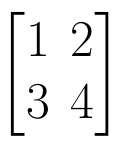. (View Answer Video)

Question 3 : If A is an invertible matrix of order 2, then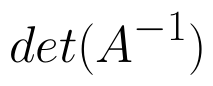is equal to, (View Answer Video)

Question 4 : If A is a symmetric matrix, then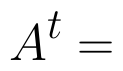? (View Answer Video)

Question 5 : If a, b, c are in AP, then determinant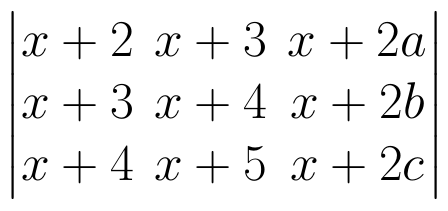. (View Answer Video)